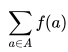# Weight Function: Definition & Examples

The weight function gives weights to data. Weights give more weight to some elements in a set.

The weight function has many uses, including:

• Compensating for bias(error),
• Giving some data points more, or less, influence. For example, you can adjust for outliers,
• Calculating integrals.

A weight function results in either a weighted mean (average) or a weighted sum.

## Weight Function Example

For example, let’s say you take three exams. The last exam is harder than the first two, so it’s given less weight. The exams are weighted as follows:

• Exam 1: 40 %
• Exam 2: 40 %
• Exam 3: 20 %

The percents listed after the tests are the weighting factors. For example, test 1 has a weighting factor of 40% while test 3 has a weighting factor of 20%. Let’s say you score 80, 80, and 85 points. The weighted mean for the three tests is found by:

1. Multiplying your scores by the percentage weights:
.4(80) = 32
.4(80) = 32
.2(95) = 19
2. Adding the numbers up. 32 + 32 + 19 = 83.

## Basic Weight Functions: Discrete Weights

Weight functions can be used for either discrete variables or continuous variables. When we are looking at a discrete data set, we define our weight function w as a positive, countable function over the set of interest, which we’ll call A. If we want all our elements to have equal weight, i.e., we want our calculations to be unweighted, the weight function becomes simply w(a) := 1.

If f is any real valued function on f, we know that we can calculate the unweighted sum of f on A with the basic formulaBut when, for whatever reason, we need to weight our data with a weight function w:A -> R+

our weighted sum becomesSuppose A was nonempty and finite. Then we know our unweighted mean (average) is given byThe weighted mean, or weighted average, would be given by## Reasons Behind Weight Functions

There are a number of reasons why a weight function may be used in calculus or engineering applications. This includes:

• Weighting for accuracy: giving greater weight to more accurate measurements and less weight to measurements known to be less precise.
• Compensating for bias: giving greater weight to measurements known to be less biased.
• Accounting for significance: in engineering applications, a weighting function may simply reflect the relative influence of various forces being applied.

References:
European Nuclear Society. Tissue Weighting Factor. Retrieved 9/20/2006 from: https://www.euronuclear.org/info/encyclopedia/t/tissue-weight-factor.htm
International Commission on Radiological Protection. The 2007 Recommendations of the International Commission on Radiological Protection. ICRP publication 103. Ann ICRP. 2007;37 (2-4): 1-33. Available from PubMed.